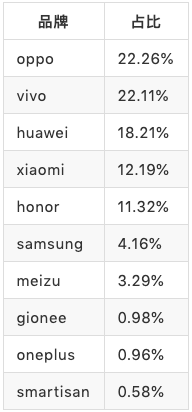## 0x01 设备指纹

• 硬件环境 （cpu型号，主板型号，制造商等...）

• 硬件运行的环境（充电，电量，罗盘等...）

• 软件环境（系统版本，组件版本等...）

• 软件运行的环境（内存，写入速度，运行速度等...）

## 0x02 特征工程和建模• TOP 10的占比情况``Text(1.1,-0.000515123,"ztev987"), ``Text(1.1,-0.000513474,"f8"), ``Text(1.1,-0.000511826,"zte-e3"), ``Text(1.1,-0.000510177,"f8909"), ``Text(1.1,-0.000508529,"f8919"), ``Text(1.1,-0.000506881,"定制版"), ``Text(1.1,-0.000505232,"小米mix"), ``Text(1.1,-0.000503584,"desay"), ``Text(1.1,-0.000501935,"翼触"), ``Text(1.1,-0.000500287,"鱿鱼客"), ``Text(1.1,-0.000498639,"dostyle"), ``Text(1.1,-0.00049699,"韩众"), ``Text(1.1,-0.000495342,"青葱metal"), ``Text(1.1,-0.000493694,"长虹"), ``Text(1.1,-0.000492045,"迪美"), ``Text(1.1,-0.000490397,"谷歌"), ``Text(1.1,-0.000488748,"dow"), ``Text(1.1,-0.0004871,"e6"), ``Text(1.1,-0.000485452,"苹果"), ``Text(1.1,-0.000483803,"e889"), ``Text(1.1,-0.000482155,"美沃"), ``Text(1.1,-0.000480507,"建议该项值修改为 oppo"), ``Text(1.1,-0.000478858,"纽麦"), ``Text(1.1,-0.00047721,"e9003"), ``Text(1.1,-0.000475561,"diskon"), ``Text(1.1,-0.000473913,"王者"), ``Text(1.1,-0.000472265,"eamey"), ``Text(1.1,-0.000470616,"火米"), ``Text(1.1,-0.000468968,"detel"), ``Text(1.1,-0.00046732,"智先锋"), ``Text(1.1,-0.000465671,"edward"), ``Text(1.1,-0.000464023,"惠普"), ``Text(1.1,-0.000462374,"恒语"),``

• brand_pop

• brand_zh

• brand_symble

• brand_len

# 0x03 建模

• 分散性聚类(kmeans)

• 结构性聚类(层次聚类AGNES)

• 密度聚类(DBSCAN）

``estimator = KMeans(n_clusters=2,) #设定分类数量``estimator.fit(X) #训练``

http://scikit-learn.org/stable/modules/generated/sklearn.cluster.KMeans.html#sklearn.cluster.KMeans

• random_state 这个是控制随机种子的参数，为了让实验的变量是可控的，我们这边随机设置了一个整数

• n_init：这个是计算初始化质心的计算次数，默认是10，但是我们可以稍微调大一点，来获取更好的质心来进行分类

• max_iter：默认是300，

• tol: 与inertia结合来确定收敛条件，默认值= 1e-4

• labels_ : 各个标签的预估值

• inertia_ : 每个点到其簇的质心的距离之和 越小越好

``from sklearn.cluster import KMeans``X = trandata.values #聚类数据``estimator = KMeans(n_clusters=2,n_init=100,random_state=1,n_jobs=-1,max_iter=300,tol=0.0001) #构造聚类器``estimator.fit(X) #聚类``label_pred = estimator.labels_ #获取聚类标签 ``#获取k-means结果``x0 = X[label_pred == 0]``x1 = X[label_pred == 1]``

# 0x04 评估

F1 同时评估了 召回率和精准率 ，代码如下

``from sklearn.metrics import classification_report,precision_score,f1_score ``##表示真实的情况 ``act_y = all_data["act_y"].values``##表示预测后的值pred_y = all_data["pred_y"].values ``f1_score(act_y, pred_y, average="macro")``

``Score = (y1*f1_1 + y2*f1_2+y3*f1_3)/3``